# Quiz 18: Structuring Real Estate Investments: Organizational Forms and Joint Ventures

Business

ABC fund and NDI industry comes together to make a joint venture for new project. The initial investment requires for the joint venture project is \$100 million to cover all development cost. ABC fund contribute \$55 million and NDI industry contribute remaining \$45 million to construct the joint venture. From the cash flow first of all ABC fund receive 5% operating return and then BDI industry will receive 5% operating return. After distributing the operating return from the remaining cash flow both party distribute 50:50. After 5 year the property will sold foe \$150 million. Cash flow from project is shown below in table: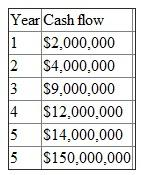As it is quoted that Investment of NDI is \$55 million. Which include NDI fee of \$50 million. Therefore, net investment by NDI is \$55million - \$50 Million = \$5 million. First of all, ABC fund will get 5% operating return then NDI will get 5% operating return then remaining amount will be distributed in 50: 50 ratios.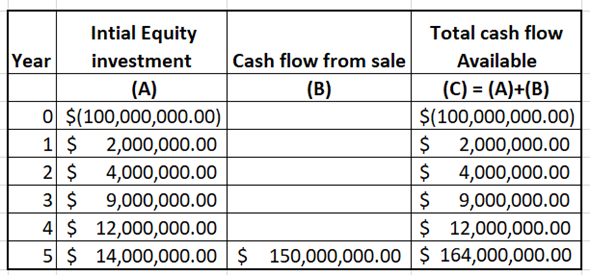For the first year and second year NDI will not receive any cash flow. During year 3, total cash available to distribution increases the to \$9,000,000. Therefore, ABC will receive 5% on its investment and NDI will also receive 5% return on its investment. The remining cash flow after distribution will be distributed in 50: 50 ratio. calculated as follows: 5% of ABC fund operating return is calculated below: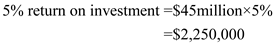5% of NDI industry operating return is calculated below: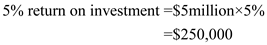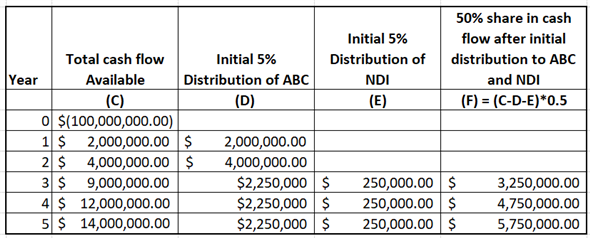ABC share of cash flow from operation is shown below: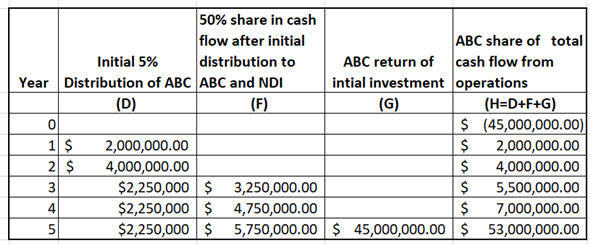To achieve 11% IRR, calculate what amount of addition cash flow is required. Let X be the additional amount.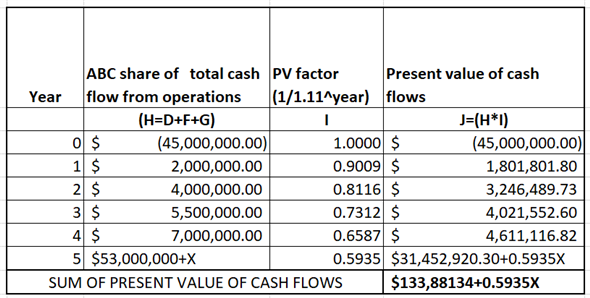SUM of present value is equal to zero to get IRR of 11%. Calculate X as follows: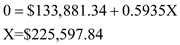Thus, Additional amount of cash flow is \$225,597.84.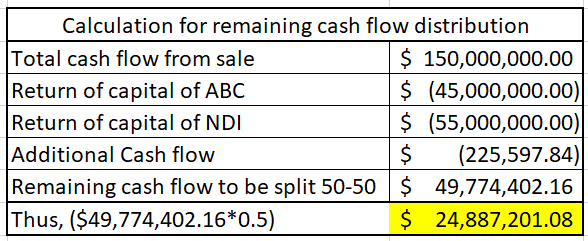Cash flows of each party is shown below. Also, Expected IRR is calculated using IRR function of excel as follows: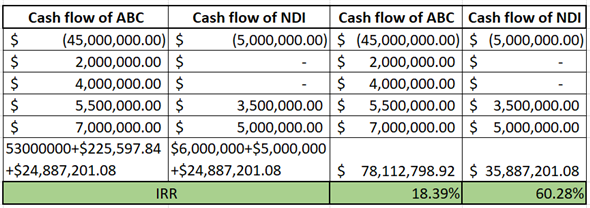Thus, expected IRR of ABC is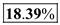and NDI is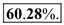.

After a property is finally sold, cash distributions from available cash flows from sales are made after debt repayment. Investors are paid off initially an amount equal to the capital invested by them in the venture. Any remaining cash flows are then distributed in proportion of amount invested by each investor. An IRR preference refers to the later part of cash distributions. Essentially it means that one or more investors will receive an amount which is sufficient to achieve the required IRR on equity invested for the entire investment period. This amount is received by investors after initial distributions are made. The additional cash flows available after distributing the IRR preference is then distributed among remaining partners in predetermined proportion for eg. 25% or 50% to each party. IRR Lookback refers to cash distribution after the initial distributions to each partner in predetermined proportion subject to the condition that one or more investor must achieve a specified IRR. If this is not achieved then some of the cash which had been previously distributed to all partners must be redistributed so that partners who must earn an IRR lookback are able to achieve their specified return. (For deeper understanding, refer to the example given under textbook explanation of the above topic.)

a. Compute ATIRR for Limited Partners in the following steps. First step: Use spreadsheet for the required computations. Enter given values and formulas in spreadsheet to create input section as shown in the image below.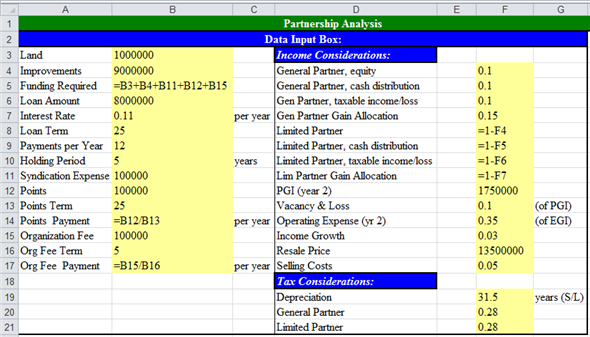Obtained results are shown below.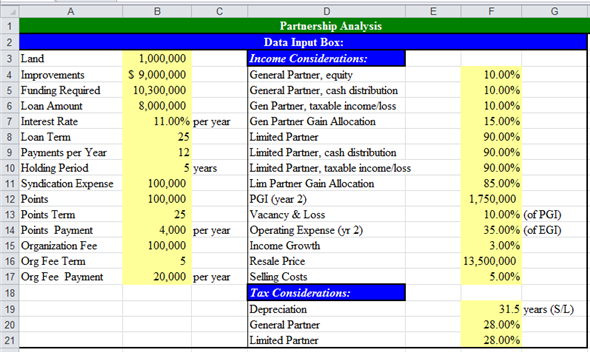Second step: Prepare summary of equity information. Enter given values and formulas in spreadsheet as shown in the image below.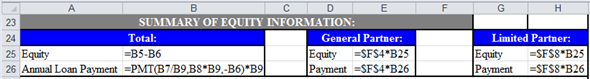Obtained results are shown below.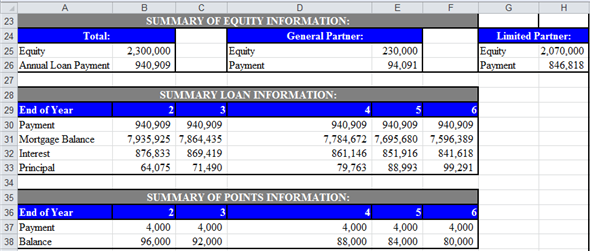Third step: Prepare summary of loan information. Enter given values and formulas in spreadsheet (in Cells A28:F33) as shown in the image below. In Cells A28:B33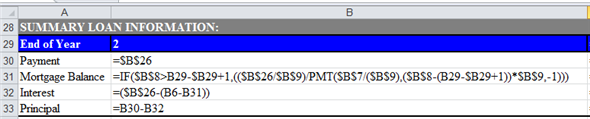In Cells C28:C33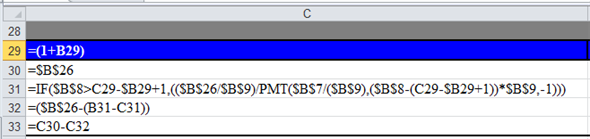In Cells D28:D33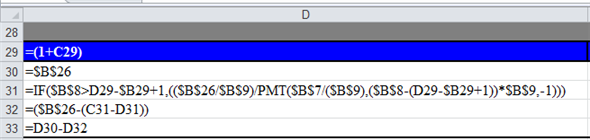In Cells E28:E33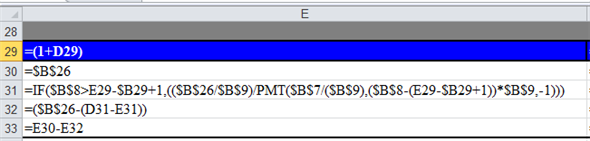In Cells F28:F33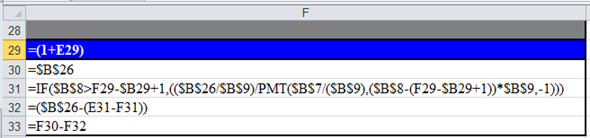Obtained results are shown below.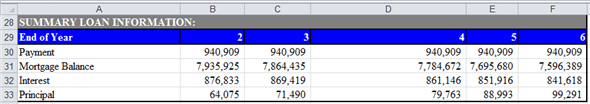Fourth step: Prepare summary of points information. Enter given values and formulas in spreadsheet as shown in the image below.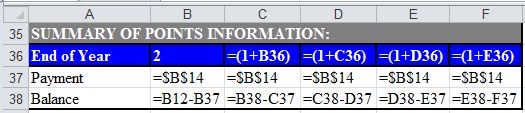Obtained results are shown below.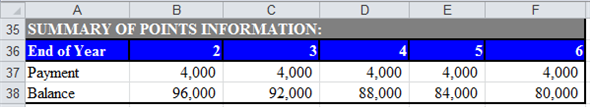Fifth step: Prepare statement of distribution of cash flows. Enter given values and formulas in spreadsheet as shown in the image below.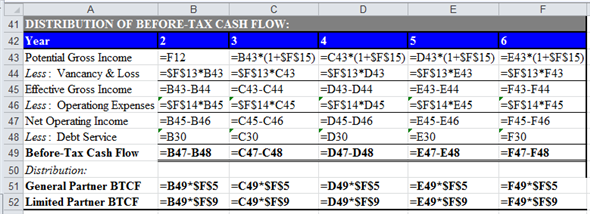Obtained results are shown below.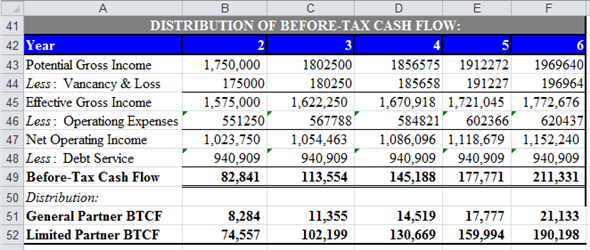Sixth step: Prepare statement of distribution of taxable income. Enter given values and formulas in spreadsheet as shown in the image below.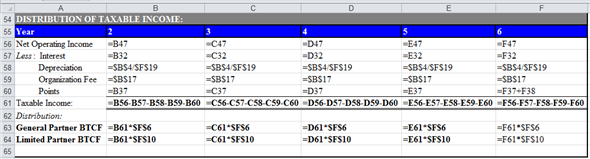Obtained results are shown below.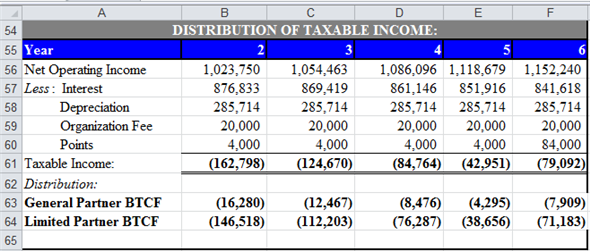Seventh step: Prepare statement of distribution of taxable gain from sale. Enter given values and formulas in spreadsheet as shown in the image below.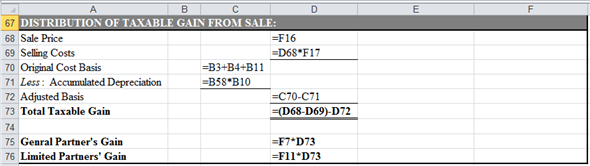Obtained results are shown below.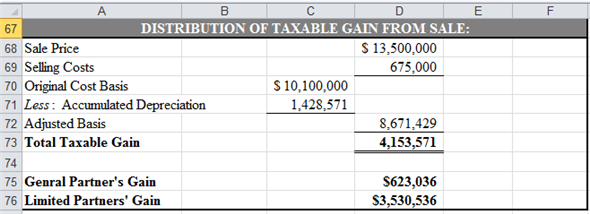Eighth step: Prepare statement of capital accounts prior to distribution of cash flow from sale. Enter given values and formulas in spreadsheet as shown in the image below.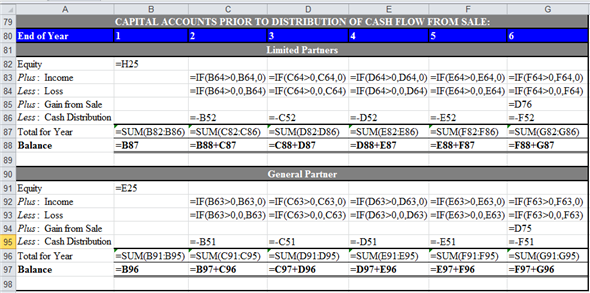Obtained results are shown below.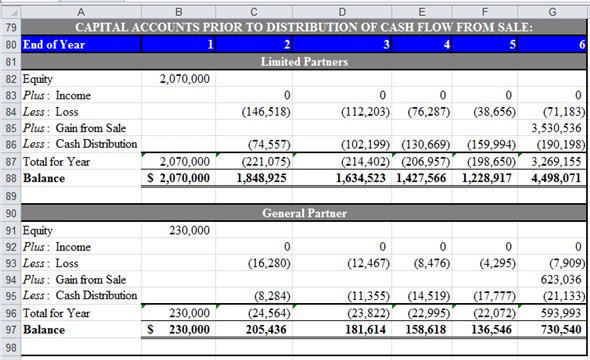Final step: Compute ATIRR (after Tax Internal Rate of Return) for Limited Partner. Enter given values and formulas in spreadsheet as shown in the image below.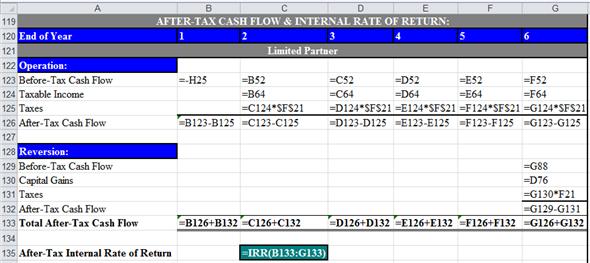Obtained results are shown below.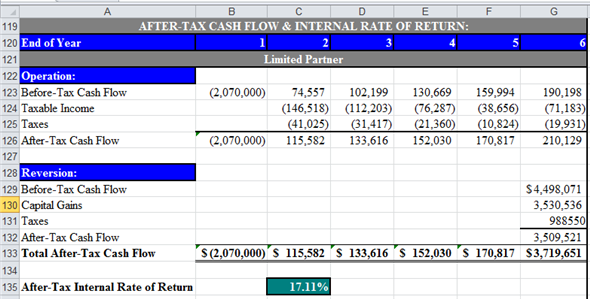Thus, the ATIRR to Limited Partner is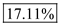. b. Compute ATIRR (after Tax Internal Rate of Return) for General Partners. Enter given values and formulas in spreadsheet as shown in the image below.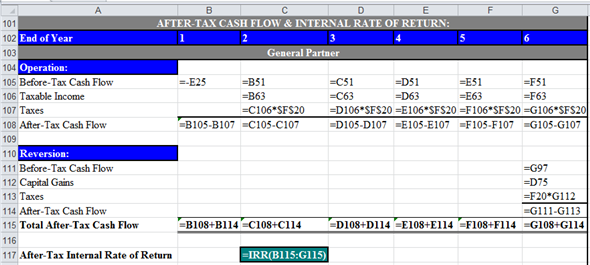Obtained results are shown below.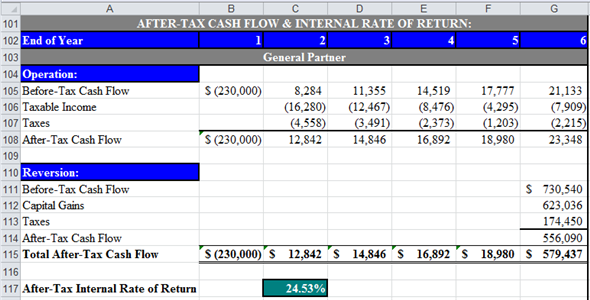Thus, the ATIRR to General Partner is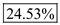. c. The ATIRR for the General Partner is the highest. This difference has occurred due the higher percentage of allocation of gain from sale to General Partners'. If the percentage of allocation of gain from the sale would have been equal to the percentage of equity contribution, then the ATIRR would have been the same for both types of partners'.